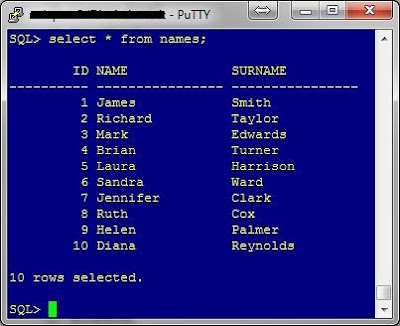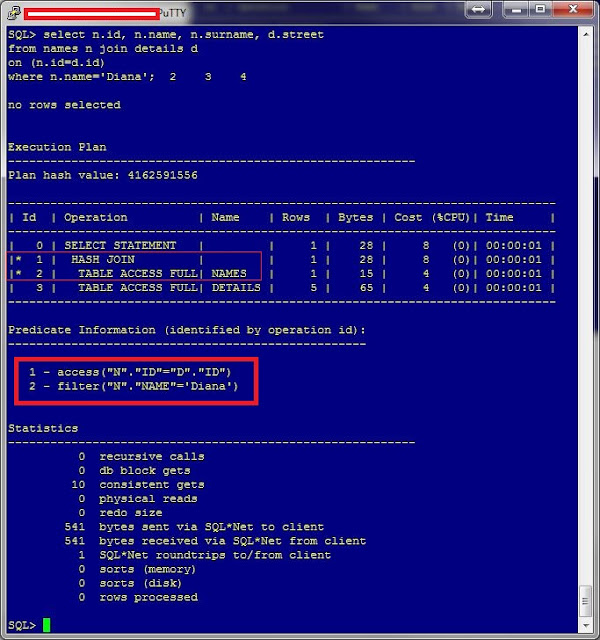## Translation

The oldest posts, are written in Italian. If you are interested and you want read the post in English, please use Google Translator. You can find it on the right side. If the translation is wrong, please email me: I'll try to translate for you.

## mercoledì, marzo 22, 2017

### Access before the filter

This post is about what happens during anti-join operation.

Usually, when there is a JOIN+FILTER, the FILTER is made before the JOIN (the access). This is because in this way the CBO can JOIN only a subset of rows of a table with another one.

But there is a case when the FILTER is applied after the ACCESS.

In this post, I show you when it happens.

For this example, I created 2 tables. Here you can find the script for creating and populating them.

These are their contents:NAMES tableDETAILS table

The ID column is the one I use in order to join the tables.

Well. What happens when I make a join between those two tables?

select
n.id
, n.name
, n.surname
, d.street
from names n
join details d
on (n.id = d.id);

Here it is.Join 01

Now I add a filter to my query

select
n.id
, n.name
, n.surname
, d.street
from names n
join details d
on (n.id = d.id)
where n.name='Diana';

This is the execution planJoin 02

This is exactly what I aspect. The filter is before of the access (the join).

In "Join 02" picture, the CBO apply the filter to the NAMES table and then make the join. Obviously, the access is made through the "ID" columns of both tables.

For my goal, I change a little the where condition:

select
n.id
, n.name
, n.surname
, d.street
from names n
join details d
on (n.id = d.id)
where d.street is NULL;

This is the execution planJoin 03

As usual, the filter is applied before the join (the access). The steps are applied in this order:
1. FILTER (id 2 of execution plan) => D.STREET IS NULL
2. ACCESS (id 1 of execution plan) at row 1 => N.ID=D.ID

At this point I re-run all previous statements, using a (LEFT) OUTER JOIN

select
n.id
, n.name
, n.surname
, d.street
from names n
left join details d
on (n.id = d.id);Join 04

The difference between the select, are;
1. Join 01: ACCESS => N.ID=D.ID
2. Join 04: ACCESS => N.ID=D.ID (+)

Using the filter....

select
n.id
, n.name
, n.surname
, d.street
from names n
left join details d
on (n.id = d.id)
where n.name='Diana';Join 05

... the ACCESS and the FILTER are the same (more or less)

1. Join 02: ACCESS => N.ID=D.ID    / FILTER => N.NAME='DIANA'
2. Join 05: ACCESS => N.ID=D.ID(+) / FILTER => N.NAME='DIANA'

For the last step, I apply the filter:

select
n.id
, n.name
, n.surname
, d.street
from names n
left join details d
on (n.id = d.id)
where d.street is NULL;Join 06

Here is the point. How you can see in this last case, the access (the join) is before the filter. It is the opposite of the Join 03. This is because now we make an ANTI (OUTER) JOIN. This is the order of operations:
1. ACCESS, (id 2 of execution plan) => N.ID=D.ID(+)
2. FILTER, (id 1 of execution plan) => D.STREET IS NULL

## mercoledì, marzo 15, 2017

### vmstat: average output

The OraPub community is very active. We chat via slack.

Someone asked a script, in order to average the output of vmstat command.

In order to do this, I made a python script. You can find it, here.

You can use it in tow ways.
1. Save the output of vmstat into a file, then use my script
2. Run my script interactively

• Save the output of vmstat into a file, then use my script

i. run vmstat and save the output
oracle> vmstat 5 5 > vmstat out

ii. Use my script
oracle> cat vmstat.out|vmstat.py
============
Samples: 4
============
Avg Proc   : 30.2 (Sum=121, Min=18, Max=37)
Avg User   : 19.0 (Sum=76, Min=18, Max=20)
Avg System : 10.8 (Sum=43, Min=10, Max=11)
Avg Idle   : 67.2 (Sum=269, Min=65, Max=68)
Avg Waiting:  3.0 (Sum=12, Min=1, Max=5)
Avg Stolen :  0.0 (Sum=0, Min=0, Max=0)

• Run my script interactively

i. run vmstat and pipe my script

oracle> vmstat 5 5 |vmstat.py
============
Samples: 4
============
Avg Proc   : 46.5 (Sum=186, Min=22, Max=61)
Avg User   : 22.5 (Sum=90, Min=21, Max=25)
Avg System : 14.8 (Sum=59, Min=14, Max=16)
Avg Idle   : 57.2 (Sum=229, Min=49, Max=60)
Avg Waiting:  5.2 (Sum=21, Min=3, Max=10)
Avg Stolen :  0.0 (Sum=0, Min=0, Max=0)

It works on Linux, Solaris, AIX and HP-UX.

I also made a script that read the vmstat output from the Oracle OS Watcher. Here an example

oracle> cat myserver_vmstat_17.03.15.1300.dat |osWatchVmstat.py
============
Samples: 162
============
Avg Proc   : 40.2 (Sum=6511, Min=37, Max=37)
Avg User   : 14.9 (Sum=2420, Min=22, Max=22)
Avg System :  8.2 (Sum=1336, Min=16, Max=16)
Avg Idle   : 71.4 (Sum=11570, Min=41, Max=41)
Avg Waiting:  5.4 (Sum=868, Min=22, Max=22)
Avg Stolen :  0.0 (Sum=0, Min=0, Max=0)

where "myserver_vmstat_17.03.15.1300.dat" is the output from OS Watch

## giovedì, marzo 02, 2017

### HINTs from V\$SQL_PLAN

In this post I want to understand how to extract the hint, querying the V\$SQL_PLAN.

The select I use, have to return just one row, otherwise

If it return more than one row, then you have the following exception
ORA-01427: single-row subquery returns more than one row

If it return NULL, then you have the following exception
ORA-06502: PL/SQL: numeric or value error
ORA-06512: at "SYS.XMLTYPE", line 272
ORA-06512: at line 1

Investigating SQL_ID= '93hwgvnjag3sz', I have more than 1 row in V\$SQL_PLAN

select SQL_ID,CHILD_NUMBER,OTHER_XML
from v\$sql_plan
where sql_id ='93hwgvnjag3sz';

For this reason, I have to modify the statement as

select other_xml
from gv\$sql_plan
where sql_id       = '93hwgvnjag3sz'
and child_number = 0
and other_xml    is not null;

In order to understand how this xml is composed, I manually format the output

So I have two sections: "other_xml" and "outline_data". Because I want to get just the hints, I rewrite the query as

select xmltype(other_xml).extract('/other_xml/outline_data')
from v\$sql_plan
where sql_id       = '93hwgvnjag3sz'
and child_number = 0
and other_xml    is not null;

and if I reformat the output ......

It's not enough, I have to go down another level. To do this I rewrite the query

select xmltype(other_xml).extract('/other_xml/outline_data/hint')
from v\$sql_plan
where sql_id       = '93hwgvnjag3sz'
and child_number = 0
and other_xml    is not null;

Because I want to see the output in better way, I use XMLTABLE function

select *
from xmltable ('/*' passing (
select xmltype(other_xml).extract('/other_xml/outline_data/hint')
from v\$sql_plan
where sql_id       = '93hwgvnjag3sz'
and child_number = 0
and other_xml    is not null)
);

XMLTABLE function, change the output of xml in an "xml table" (I don't know if it's correct) and the output is well formatted. In order to simplify the statement, I move the logic into outer select.

select *
from xmltable ('/other_xml/outline_data/hint' passing (
select xmltype(other_xml)
from v\$sql_plan
where sql_id     = '93hwgvnjag3sz'
and child_number = 0
and other_xml    is not null)
);

(obviously, the output is the same). At this point, I extract the hints from the XML

select extractvalue(value(xmltab), '.') hints
from xmltable ('/other_xml/outline_data/hint' passing (
select xmltype(other_xml)
from v\$sql_plan
where sql_id     = '93hwgvnjag3sz'
and child_number = 0
and other_xml    is not null)
) xmltab;

Cool!!!